×#### Thank you for registering.

One of our academic counsellors will contact you within 1 working day.

Click to Chat

1800-1023-196

+91-120-4616500

CART 0

• 0

MY CART (5)

Use Coupon: CART20 and get 20% off on all online Study Material

ITEM
DETAILS
MRP
DISCOUNT
FINAL PRICE
Total Price: Rs.

There are no items in this cart.
Continue ShoppingLe Chatelier’s Principle

Table of Content

Introduction to Le Chatelier’s Principle

Effect of Change of Concentration on Equilibrium

Effect of Chenage of Temperature on Equilibrium

Effect of Change of Pressure on Equilibrium

Effect of Addition of Inert Gases to a Reaction at Equilibrium

Effect of Catalyst on Equilibrium

Related Resources

Introduction to Le Chatelier’s Principle“When an equilibrium is subjected to either a change in concentration, temperature or, in external pressure, the equilibrium will shift in that direction where the effects caused by these changes are nullified”.

Refer to the following Video for Le Chatelier’s Principle

This can be understood by the following example. Overall we can also predict the direction of equilibrium by keeping in mind following theoretical assumption.

PCl5  ——> PCl3 + Cl2

Let us assume that we have this reaction at equilibrium and the moles of Cl2, PCl3 and PCl5 at equilibrium are a, b and c respectively, and the total pressure be PT.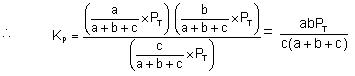Since

PT  =  (a+b+c) RT / V

KP = abRT / cV

Now if d moles of PCl3 is added to the system, the value of Q would be, a(b+d)RT / cV

We can see that this is more than KP. So the system would move reverse to attain equilibrium.

If we increase the volume of the system, the Q becomes  abRT / cV' where V' > V. Q becomes less, and the system would move forward to attain equilibrium.

If we add a noble gas at constant pressure, it amounts to increasing the volume of the system and therefore the reaction moves forward.

If we add the noble gas at constant volume, the expression of Q remains as Q = abRT / cV and the system continues to be in equilibrium. Nothing happens.

Therefore for using Le-Chatlier’s principle, convert the expression of KP and KC into basic terms and then see the effect of various changes.

Effect of Change of Concentration on Equilibrium

Let us have a general reaction,

aA + bB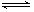cC + dD

at a given temperature, the equilibrium constant,

Kc = [C]c[D]d / [A]a[B]b

again if α, β, γ and δ are the number of mole of A, B, C and D are at equilibrium

then, Kc = [γ]c[δ]d / [α]a[β]b

If any of product will be added, to keep the Kc constant, concentration of reactants will increase i.e. the reaction will move in reverse direction. Similarly if any change or disturbance in reactant side will be done, change in product’s concentration will take place to minimise the effect.

Effect of Chenage of Temperature on Equilibrium

The effect of change in temperature on an equilibrium cannot be immediately seen because on changing temperature the equilibrium constant itself changes. So first we must find out as to how the equilibrium constant changes with temperature.

For the forward reaction, according to the Arrhenius equation,And for the reverse reaction,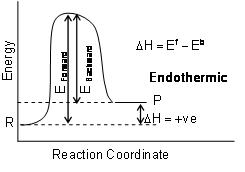R = Reactant, P = Product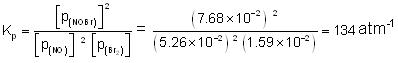It can be seen thatFor any reaction, ΔH = Eaf – EarFrom the equation,

logK2/K1 = ΔHo / 2.303R (1/T1 – 1/T2) it is clear that

(a) If ΔHo is +ve (endothermic), an increase in temperature (T2 > T1) will make K2 > K1, i.e., the reaction goes more towards the forward direction and vice-versa.

(b) If ΔHo is -ve (exothermic), an increase in temperature (T2 > T1), will make K2 < K1 i.e., the reaction goes in the reverse direction.

Increase in temperature will shift the reaction towards left in case of exothermic reactions and right in endothermic reactions.

Increase of pressure (decrease in volume) will shift the reaction to the side having fewer moles of the gas; while decreases of pressure (increase in volume) will shift the reaction to the side having more moles of the gas.

If no gases are involved in the reaction higher pressure favours the reaction to shift towards higher density solid or liquid.

Refer to the following Video for Arrhenius equation

Example . Under what conditions will the following reactions go in the forward direction?

(i)  N2(g)+ 3H2(g)2NH3(g) + 23 k cal.

(ii) 2SO2(g) + O2(g)2SO3(g) + 45 k cal.

(iii) N2(g) + O2(g)2NO(g) - 43.2 k cal.

(iv) 2NO(g) + O2(g)2NO2(g) + 27.8 k cal.

(v) C(s) + H2O(g)CO2(g) + H2(g) + X k cal.

(vi) PCl5(g)PCl3(g) + Cl­2(g)- X k cal.

(vii) N2O4(g)2NO2(g) - 14 k cal.

Solution:

(i)  Low T, High P, excess of N2 and H2.

(ii) Low T, High P, excess of SO2 and O2.

(iii) High T, any P, excess of N2 and O2

(iv) Low T, High P, excess of NO and O2

(v) Low T, Low P, excess of C and H2O

(vi) High T, Low P, excess of PCl5

(vii) High T, Low P, excess of N2O4.

Example: The equilibrium constant KP for the reaction N2(g) + 3H2(g)2NH3(g)  is 1.6 × 10-4atm-2 at 400oC. What will be the equilibrium constant at 500oC  if heat of the reaction in this temperature range is-25.14 k cal?

Solution: Equilibrium constants at different temperature and heat of the reaction are related by the equation,log KP2 = –25140 / 2.303 × 2 [773 – 673 / 773 × 673]  + log 1.64 10-4

log KP2 = –4.835

KP2 = 1.462 × 10–5 atm–2

Effect of Change of Pressure on Equilibrium

The effect of change of pressure on chemical equilibrium can be done by the formula.

Kp = QP × P(Δn)

Case A: When Δn = 0

Kp = Qp

And equilibria is independent of pressure.

Case B: When Δn = – ve

Kp = QP × P–Δn = QP / PΔn

Here with increase in external pressure, will shift the equilibrium towards forward direction to maintain K­p. Similarly, decrease in pressure will shift the equilibrium in the reverse direction.

Case C: When Δn = +ve

Kp = QP × P–Δn and, effect will be opposite to that of Case ‘B’

Effect of Addition of Inert Gases to a Reaction at Equilibrium

Let us take a general reaction

aA + bBcC + dD

We know,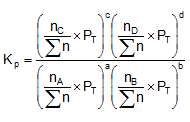Where,

nC nD, nA, nB denotes the no. of moles of respective components and PT is the total pressure and n = total no. of moles of reactants and products.

Now, rearranging,Where n = (c + d) – (a + b)

Now, n can be = 0, < 0 or > 0

Lets take each case separately.

a)   n = 0 : No effect

b)   n = ‘+ve’ : Addition of inert gas increases the  n i.e. is decreased and so is . So products have to increase and reactants have to decrease to maintain constancy of Kp. So the equilibrium moves forward.

c) n = ‘–ve’  In this case  decreases but increases. So products have to decrease and reactants have to increase to maintain constancy of Kp. So the equilibrium moves backward.

2. Addition at Constant Volume : Since at constant volume, the pressure increases with addition of inert gas and at the same time ån also increases, they almost counter balance each other. So  can be safely approximated as constant. Thus addition of inert gas has no effect at constant volume.

Effect of Catalyst on Equilibrium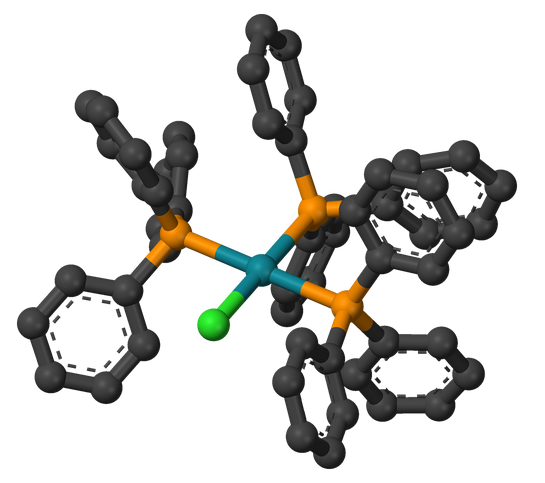Catalysts are the substances which  alter the rate of a reaction without themselves getting consumed in the reaction.  Since the catalyst is associated with forward as well as reverse direction reaction. So, at equilibrium, rate of forward reaction will be equal to rate of reverse reaction and hence catalyst effect will be same on both forward as well as reverse. Hence catalyst never effect the point of equilibrium but it reduces the time to attain the equilibrium.Example: What will be the effect on the equilibrium constant on increasing the temperature.

Solution: Since the forward reaction is endothermic, so increasing the temperature, the forward reaction is favoured. Thereby the equilibrium constant will increase.

Sample problem Kp for the reaction 2BaO2(s)2BaO(s) + O2(g) is 1.6 × 10–4 atm, at 400°C. Heat of reaction is – 25.14 kcal. What will be the no. of moles of O2 gas produced at 500°C temperature, if it is carried in 2 litre reaction vessel?

Solution:   We know that

log LP2/KP1 = ΔH / 2.303R [1/T1 – 1/T2]

log KP2/1.6 × 10–4 = –25.14 / 2.330 × 2 × 10–3 [773 – 673 / 773 × 673]

=> KP2/1.46 × 10–5 atm

KP2 = p02 = 1.46 × 10–5 atm

Since, PV = nRT

1.46 × 10–5 × 2 = n × 0.0821 × 773

n = 1.46 × 10–5 / 0.0821 × 773 = = 4.60 × 10-7

Related Resources

Chemistry Syllabus for JEE

Reference books of Chemistry

To read more, Buy study materials of Chemical Equilibrium comprising study notes, revision notes, video lectures, previous year solved questions etc. Also browse for more study materials on Chemistry here.### Course Features

• 731 Video Lectures
• Revision Notes
• Previous Year Papers
• Mind Map
• Study Planner
• NCERT Solutions
• Discussion Forum
• Test paper with Video Solution